# SAT Math Multiple Choice Question 49: Answer and Explanation

### Test Information

Question: 49

5.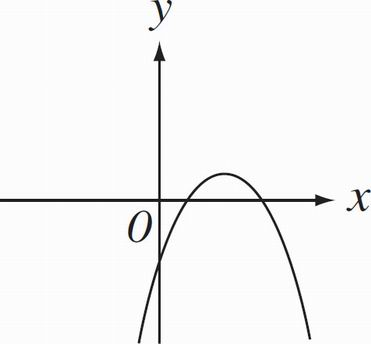The graph of y = g(x) is shown in the figure above. If g(x) = ax2 + bx + c for constants a, b, and c, and if abc ≠ 0, then which of the following must be true?

• A. ac > 1
• B. c > 1
• C. ac > 0
• D. a > 0

C Remember your transformation rules. Whenever a parabola faces down, the quadratic equation has a negative sign in front of x2 term. It always helps to plug in! Let's take an example. If your original equation was (x - 2)2, putting a negative sign in front would make the parabola open downward, so you'll have -(x - 2)2. If you expand it out, you get -x2 + 4x - 4. Notice that the value of a in this equation is -1. Also notice that the value of c is -4. This allows you to eliminate (B) and(D). Now you must plug in differently to distinguish between (A) and (C). Be warned: You must use fractions to help discern which is correct. Let's say the x-intercepts take place at x =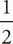and x = x =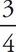. Rewriting those two expressions means that the factors are and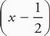and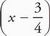. If you FOIL out the terms, you end up with x2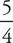- x +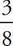. Remember, the parabola opens downward, so you must multiply by -1 to each term to yield -x2 +x -. Your values of a and c are now -1 and -, respectively. Multiply the two values and you get, which allows you to eliminate (A) and confidently select (C). (And that was worth only 1 point! Embrace the POOD!)